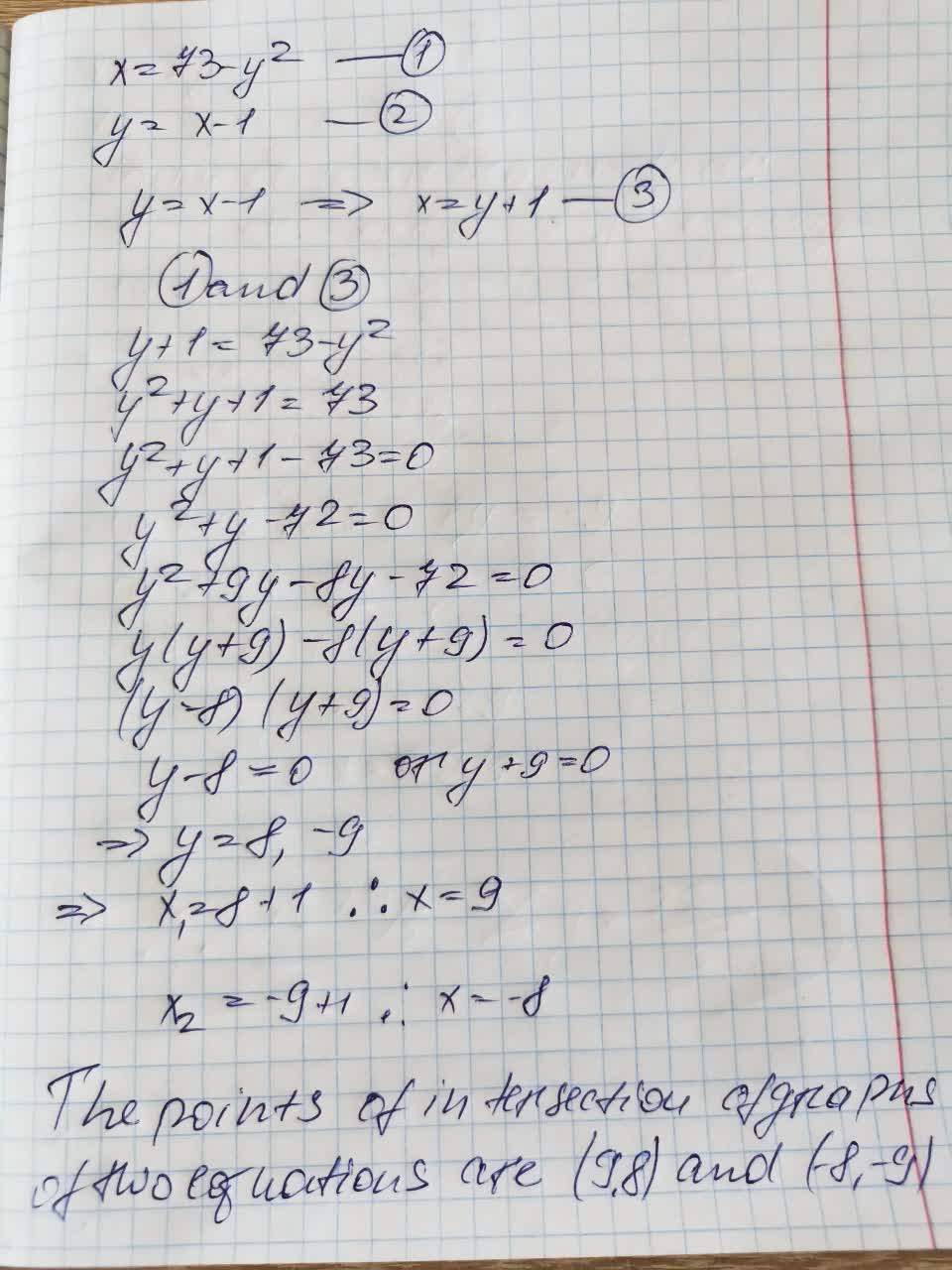# You can find the point(s) of intersection of the graphs of the given equations by solving their equations simultaneously.Solve the first equation forKhadija Wells 2021-09-04 Answered

Find the point(s) of intersection of the graphs of the equations.

$x=73-{y}^{2}$

$y=x-1$

A point of intersection of the graphs of two equations is a point that satisfies both the equations. You can find the point(s) of intersection of the graphs of the given equations by solving their equations simultaneously.

Solve the first equation for ${y}^{2}$.

$x=73-{y}^{2}$

$={y}^{2}$

You can still ask an expert for help

• Questions are typically answered in as fast as 30 minutes

Solve your problem for the price of one coffee

• Math expert for every subject
• Pay only if we can solve itrogreenhoxa8

Explanation